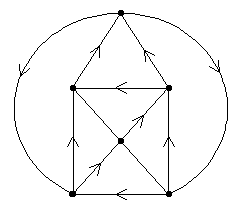## Project 3: Orienting Graphs

Let G be a k-valent graph (i.e. there are k edges at each vertex).

Problems

1. Can the edges of G be oriented so that for every pair of vertices v and w of the oriented graph outval(v) = outval (w) and inval(v) = inval(w)? If not, for what graphs can this be done? (In a directed graph outval(v) counts the number of edges which are directed away from vertex v and inval (v) counts the number of edges which point towards v.) Figure 1, for example, shows for one graph G, a way of orienting the graph so that at each vertex the invalence and outvalence are each 2.Figure 1

2. Repeat this question but in particular for plane graphs (i.e. graphs which have been drawn in the plane so edges meet only at vertices). Can the same cyclic pattern be achieved at each vertex in this case?

Extensions:

1. Are there graphs for which different orientations can be made which achieve the different possible "identical" patterns of in and out edges at each vertex? (For example, in a 4-valent graph one can have all vertices with inval =3 and outval = 1, or inval =2 and outval = 2, or inval 1 and outval 3 patterns.)

Joseph Malkevitch
Department of Mathematics and Computing
York College (CUNY)
Jamaica, New York 11451-0001
email: malkevitch@york.cuny.edu
(Comments and results related to the project above are welcome.)

Acknowledgements
Some of this work was prepared with partial support from the National Science Foundation (Grant Number: DUE 9555401) to the Long Island Consortium for Interconnected Learning (administered by SUNY at Stony Brook, Alan Tucker, Project Director).